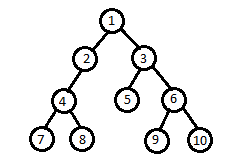New update is available. Click here to update.

# LCA of three Nodes

Last Updated: 10 Oct, 2020
Difficulty: Easy

## PROBLEM STATEMENT

#### You have been given a Binary Tree of 'N' nodes where the nodes have integer values and three integers 'N1', 'N2', and 'N3'. Find the LCA(Lowest Common Ancestor) of the three nodes represented by the given three('N1', 'N2', 'N3') integer values in the Binary Tree.

##### For example:``````For the given binary tree: the LCA of (7,8,10) is 1
``````
##### Note:
``````All of the node values of the binary tree will be unique.

N1, N2, and N3  will always exist in the binary tree.
``````
##### Input Format:
``````The first line of input contains a single integer T, representing the number of test cases or queries to be run.

Then the T test cases follow.

The first line of each test case contains three single space-separated integers N1, N2, and N3, denoting the nodes of which LCA is to be calculated.

The second line of each test case contains elements in the level order form. The line consists of values of nodes separated by a single space. In case a node is null, we take -1 in its place.

For example, the input for the tree depicted in the below image would be :
````````````1
2 3
4 -1 5 6
-1 7 -1 -1 -1 -1
-1 -1
``````

#### Explanation :

``````Level 1 :
The root node of the tree is 1

Level 2 :
Left child of 1 = 2
Right child of 1 = 3

Level 3 :
Left child of 2 = 4
Right child of 2 = null (-1)
Left child of 3 = 5
Right child of 3 = 6

Level 4 :
Left child of 4 = null (-1)
Right child of 4 = 7
Left child of 5 = null (-1)
Right child of 5 = null (-1)
Left child of 6 = null (-1)
Right child of 6 = null (-1)

Level 5 :
Left child of 7 = null (-1)
Right child of 7 = null (-1)

The first not-null node (of the previous level) is treated as the parent of the first two nodes of the current level. The second not-null node (of the previous level) is treated as the parent node for the next two nodes of the current level and so on.
The input ends when all nodes at the last level are null (-1).
``````
##### Note :
``````The above format was just to provide clarity on how the input is formed for a given tree.
The sequence will be put together in a single line separated by a single space. Hence, for the above-depicted tree, the input will be given as:

1 2 3 4 -1 5 6 -1 7 -1 -1 -1 -1 -1 -1
``````
##### Output Format:
``````For each test case,  return the node representing LCA.
``````
##### Note
``````You do not need to print anything, it has already been taken care of. Just implement the given function.
``````
##### Constraints:
``````1 <= T <= 10
1 <= N <= 10^5
0 <= node data <= 10^9
0 <= N1 <= 10^9
0 <= N2 <= 10^9
0 <= N3 <= 10^9

Time Limit: 1sec
``````## Approach 1

• A simple solution would be to store the path from the root to ‘N1’, the path from the root to ‘N2’, and the path from the root to ‘N3’ in the three auxiliary Lists/Array.
• Now, to store the path from the root to any node ‘X’ we create a recursive function that traverses the different path in the binary tree to find any node ‘X’:
1. If ‘ROOT’ == null return true
2. Add the root data to List/Array
3. If root data = ‘X’ return true
4. If ‘X’ is found in any of the subtrees either left or right then return true
5. Else remove the current root data from the List/Array and then return false
• Then we traverse all lists simultaneously till the values in the list match. The last matched value will be the LCA. If the end of one array is reached then the last seen value is LCA.